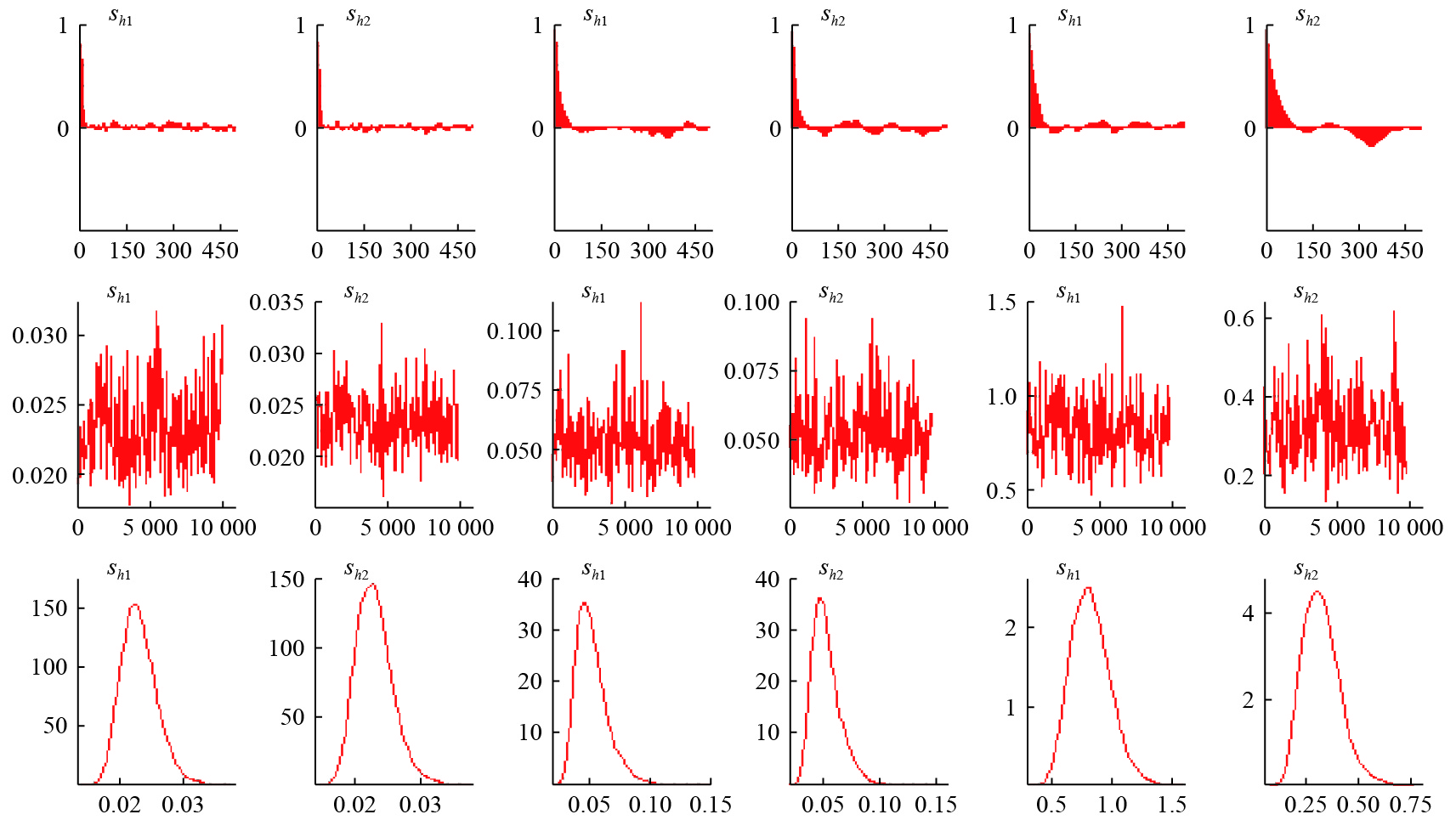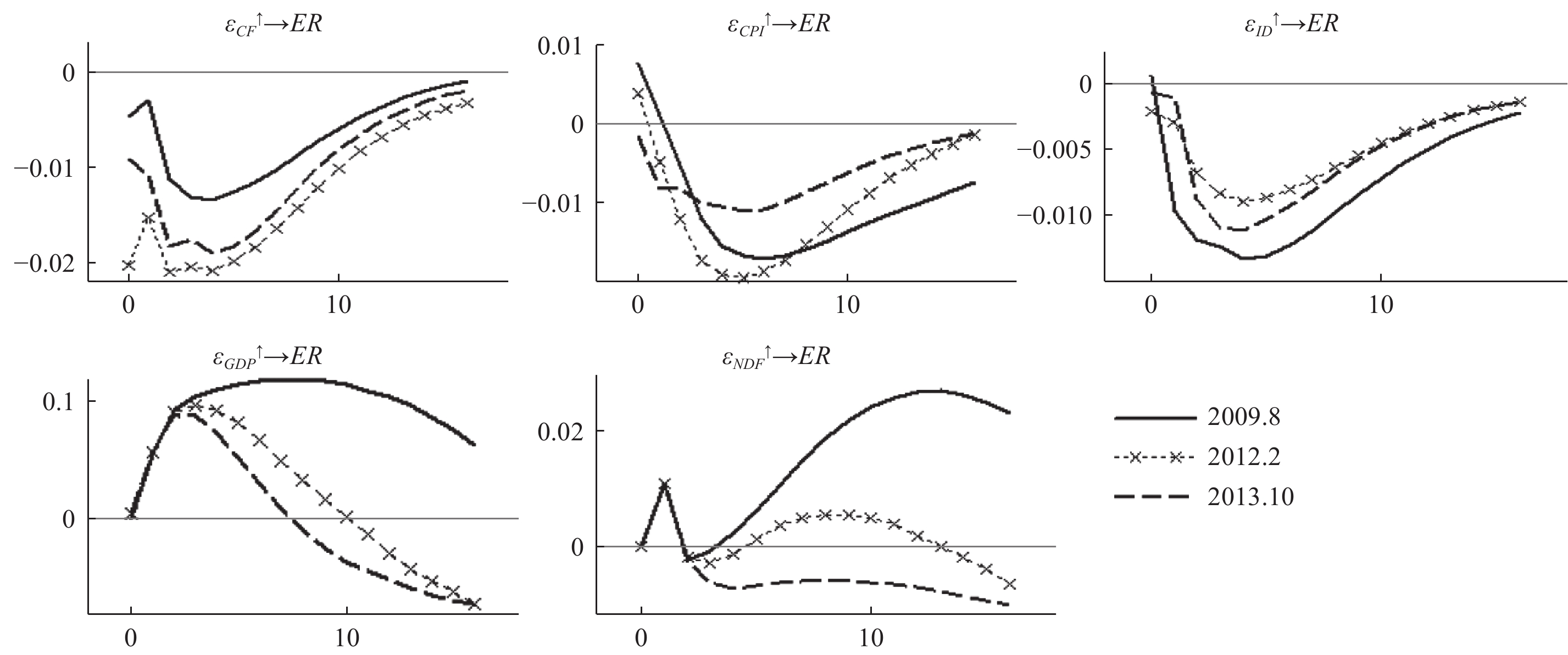﻿ 泰勒规则、国际资本流动与人民币汇率动态决定《财经研究》
2019第45卷第9期

1. 青岛大学 经济学院，山东 青岛 266061;
2. 中国海洋大学 经济学院，山东 青岛 266100;
3. 苏州大学 东吴商学院，江苏 苏州 215012;
4. 中国农业大学 经济管理学院，北京 100083

Taylor Rule, International Capital Flows and the Dynamic Determinant of RMB Exchange Rate
Si Dengkui1, Li Xiaolin2, Ge Xinyu3, Liu Zhenning4
1. School of Economics, Qingdao University, Qingdao 266061, China;
2. School of Economics, Ocean University of China, Qingdao 266100, China;
3. Dongwu Business School, Soochow University, Suzhou 215012, China;
4. College of Economics and Management, China Agricultural University, Beijing 100083, China
Summary: The report of the Nineteenth National Congress of the CPC and the 2018 Central Economic Work Conference clearly pointed out that " maintaining the basic stability of RMB exchange rate at a reasonable and balanced level”. Therefore, while enhancing exchange rate flexibility, maintaining the orderly fluctuation of RMB exchange rate at the level of agreed equilibrium will be an important issue to the monetary authorities. This paper takes the monetary policy of open Taylor Rule as the framework, and endogenously implants international capital flows to examine the dynamic determinant mechanism of RMB exchange rate. In particular, we choose the sample period from July 2005 to October 2018, and adopt the TVP-SV-BVAR model to take an empirical analysis from different perspectives and periods. The following results are obtained: (1) Both the inflow of international capital and the expansion of interest margin will boost the appreciation of RMB exchange rate in the short and long term, and the impact will increase significantly after the " exchange rate reform” in 2015. (2) The rise of inflation will also cause the appreciation of RMB exchange rate in the short and long term. However, this impact is relatively small in most periods. (3) Output increasing will lead to RMB devaluation in the short term, but it will promote RMB appreciation in the long term. Compared with other variables, the output gap has the greatest impact on the exchange rate, and is relatively stable in most sample periods. (4) The impact of exchange rate expectations on RMB spot exchange rate will be positive in the short term, but negative in the long term due to foreign exchange intervention. The results of this paper have important implications for preventing the abnormal flow of international capital and stabilizing the fluctuation of RMB exchange rate. In particular, the decision-making authorities should implement integrated supervision of international capital flows to avoid risks or even crises arising from large-scale capital flows. Taylor Rule, which includes international capital flows, plays an important role in promoting the marketization of the exchange rate and the interest rate. This paper expands the existing research from two aspects: Firstly, it endogenously implants international capital flows into the open Taylor Rule model and analyzes the dynamic determinant mechanism of RMB exchange rate from the theoretical level. Secondly, we utilize the TVP-SV-BVAR model to empirically analyze the nonlinear effect of Taylor Rule including international capital flows on RMB exchange rate from different points and periods. In addition, with the increasing complexity of domestic and foreign economic environment, the causes of regional heterogeneity involved in the study can be further identified.
Key words: Taylor Rule    international capital flows    exchange rate    TVP-SV-BVAR

 $i_{t}^{*}=r^{*}+\pi_{t}+\alpha_{0}\left(\pi_{t}-\pi^{*}\right)+\alpha_{2} y_{t}$ (1)

$i_t^*$
${r^*}$

${\pi _t}$
${\pi ^*}$

${y_t}$

${\alpha _0}$
${\alpha _2}$

 $i_t^* = {r^*} + {\pi _t} + {\alpha _0}({\pi _t} - {\pi ^*}) + {\alpha _2}{y_t} + {\alpha _3}c{f_t}$ (2)

$c{f_t}$

${\alpha _3}$

$\mu = {r^*} - {\alpha _0}{\pi ^*}$
${\alpha _1} = 1 + {\alpha _0}$
，同时为了将泰勒规则拓展至开放形式，我们还将汇率偏离纳入模型之中，形式变换如下：

 $i_t^* = \mu {\rm{ + }}{\alpha _1}{\pi _t} + {\alpha _2}{y_t} + {\alpha _3}c{f_t} + {\alpha _4}({e_t} - {\bar e_t})$ (3)

${e_t}$

${\bar e_t}$

${e_t} - {\bar e_t}$

 ${i_t} = \rho {i_{t - 1}} + (1 - \rho )i_t^{\rm{*}} + {\varepsilon _t}$ (4)

${\varepsilon _t}$

 ${i_t} = \rho {i_{t - 1}} + (1 - \rho )\left[ {{\alpha _1}{\pi _t} + {\alpha _2}{y_t} + {\alpha _3}c{f_t} + {\alpha _4}({e_t} - {{\bar e}_t}) + \mu } \right] + {\varepsilon _t}$ (5)

 $i_t^f = \rho i_{t - 1}^f + (1 - \rho )[{\alpha _1}\pi _t^f + {\alpha _2}y_t^f + \alpha _4^*({e_t} - {\bar e_t}) + {\mu ^f}] + \varepsilon _t^f$ (6)

 \begin{aligned} {i_t} - i_t^f =& \rho ({i_{t - 1}} - i_{t - 1}^f) + (1 - \rho ){\alpha _1}({\pi _t} - \pi _t^f) + (1 - \rho ){\alpha _2}({y_t} - y_t^f)\\ & + (1 - \rho ){\alpha _3}c{f_t} + (1 - \rho )({\alpha _4} - \alpha _4^*)({e_t} - {{\bar e}_t})\\ & + (1 - \rho )(\mu - {\mu ^f}) + {\varepsilon _t} - \varepsilon _t^f \end{aligned} (7)

${\varepsilon _t} - \varepsilon _t^f$

 ${E_t}{e_{t + 1}} = {e_t} + {i_t} - i_t^f$ (8)
 ${\bar e_t} = {p_t} - p_t^f$ (9)

${i_t}$
$i_t^f$

${p_t}$
$p_t^f$

${e_t}$

${E_t}{e_{t + 1}}$

 \begin{aligned} [1 + (1 - \rho )({\alpha _4} - \alpha _4^*)]{e_t} =& {E_t}{e_{t + 1}} - \rho ({i_{t - 1}} - i_{t - 1}^f) - (1 - \rho ){\alpha _1}({\pi _t} - \pi _t^f)\\ & - (1 - \rho ){\alpha _2}({y_t} - y_t^f) + (1 - \rho )({\alpha _4} - \alpha _4^*)({p_t} - p_t^f)\\ & - (1 - \rho ){\alpha _3}c{f_t} - (1 - \rho )(\mu - {\mu ^f}) - ({\varepsilon _t} - \varepsilon _t^f) \end{aligned} (10)

$s = \dfrac{1}{{1 + (1 - \rho )({\alpha _4} - \alpha _4^*)}}$
，并对et进行求解，可得：

 \begin{aligned} {e_t} = &s{E_t}{e_{t + 1}} - s\rho ({i_{t - 1}} - i_{t - 1}^f) - s({\varepsilon _t} - \varepsilon _t^f) - s(1 - \rho )(\mu - {\mu ^f})\\ & - s(1 - \rho )[{\alpha _1}({\pi _t} - \pi _t^f) + {\alpha _2}({y_t} - y_t^f) + {\alpha _3}c{f_t} - ({\alpha _4} - \alpha _4^*)({p_t} - p_t^f)] \end{aligned} (11)

${\pi _t} = {p_t} - {p_{t - 1}}$

 \begin{aligned} {e_t} =& s{E_t}{e_{t + 1}} - s\rho ({i_{t - 1}} - i_{t - 1}^f) - s({\varepsilon _t} - \varepsilon _t^f) - s(1 - \rho )(\mu - {\mu ^f})\\ & - s(1 - \rho )\{ [{\alpha _1} - ({\alpha _4} - \alpha _4^*)]({p_t} - p_t^f) - {\alpha _1}({p_{t - 1}} - p_{t - 1}^f) + {\alpha _2}({y_t} - y_t^f) + {\alpha _3}c{f_t}\} \end{aligned} (12)

$\mu$
${\mu ^f}$

${\varepsilon _t}$
$\varepsilon _t^f$

 τ 0.1 0.2 0.3 0.4 0.5 0.6 0.7 0.8 0.9 ER ${\alpha _1}(\tau )$ −1.133 −3.301 −4.146 −3.922 −3.818 −4.327 −3.540 −2.452 −0.259 p 0.276 0.004 0.000 0.000 0.006 0.000 0.004 0.056 0.734 QKS 9.004*** K 1 F 467.574*** CF ${\alpha _1}(\tau )$ −2.906 −2.856 −3.820 −3.742 −3.700 −3.952 −3.205 −7.537 −5.197 p 0.028 0.016 0.004 0.006 0.012 0.002 0.012 0.000 0.000 QKS 7.451*** K 2 F 19.458*** ID ${\alpha _1}(\tau )$ −5.402 −5.958 −5.362 −7.596 −10.316 −9.050 −9.308 −4.129 −2.598 p 0.002 0.000 0.000 0.000 0.000 0.000 0.000 0.002 0.048 QKS 8.726*** K 1 F 254.374*** GDP ${\alpha _1}(\tau )$ −3.772 −7.024 −5.438 −7.487 −10.113 −8.666 −7.878 −3.923 −0.824 p 0.006 0.000 0.000 0.000 0.000 0.000 0.000 0.012 0.398 QKS 6.462*** 2 F 29.387*** CPI ${\alpha _1}(\tau )$ −1.321 −1.663 −2.544 −2.900 −2.607 −2.312 −3.173 −2.596 −2.225 p 0.270 0.236 0.042 0.024 0.054 0.108 0.008 0.026 0.094 QKS 5.943* K 3 F 47.151*** NDF ${\alpha _1}(\tau )$ −1.348 −1.849 −2.439 −3.422 −3.848 −4.115 −3.129 −2.095 −0.498 p 0.292 0.206 0.068 0.006 0.000 0.000 0.012 0.128 0.730 QKS 7.427*** K 1 F 229.660*** 结构突变点位置 突变点1 突变点2 突变点3 突变点4 突变点5 ER 2008M1 2009M10 2011M9 2013M1 2015M7 CF 2012M3 2013M8 2015M7 2017M6 ID 2007M4 2008M11 2010M11 2013M4 GDP 2008M9 2010M1 CPI 2008M6 2010M11 2012M8 2013M12 2015M4 NDF 2006M12 2008M8 2011M3 2013M2 2015M7 注：***和*分别表示1%和10%的显著性水平； ${\alpha _1}(\tau )$ 表示在不同分位数水平 $\tau$ 下对变量的残差序列进行平稳性检验时的自回归系数；p 为检验 ${\alpha _1}(\tau )$ 是否显著时的伴随概率；QKS为含傅里叶级数的分位数单位根检验统计量，其原假设为变量在全局内非平稳，其临界值根据5 000次用bootstrap算法获得；K表示平滑渐变点的个数；F统计量用来检验变量是否为线性形式，原假设为“变量为线性”，此时拟合变量数据生成过程的傅里叶级数中的正弦(sine)与余弦(cosine)项均不显著。

（一）参数估计

 参数 均值 标准差 95%置信区间 CD检验 无效因子 ${\left( {\sum\nolimits_\beta } \right)_1}$ 0.0232 0.0027 [0.0186,0.0291] 0.1320 13.09 ${\left( {\sum\nolimits_\beta } \right)_2}$ 0.0230 0.0028 [0.0183,0.0292] 0.7810 8.86 ${\left( {\sum\nolimits_\alpha } \right)_1}$ 0.0525 0.0129 [0.0339,0.0834] 0.7510 25.34 ${\left( {\sum\nolimits_\alpha } \right)_2}$ 0.0523 0.0125 [0.0338,0.0818] 0.2480 25.51 ${\left( {\sum\nolimits_h {} } \right)_1}$ 0.8297 0.1583 [0.5572,1.1742] 0.5010 35.82 ${\left( {\sum\nolimits_h {} } \right)_2}$ 0.3240 0.0874 [0.1833,0.5237] 0.3460 54.62 注：α、β和h (i, j, k= 1, 2, 3)分别表示Σα、Σβ和Σh的第i、j和k个对角元素，并且Σα和Σβ的估计值和标准差都乘以100。CD检验为Geweke (1992)提出的收敛性检验（Convergence diagnostics），其原假设为参数收敛于后验分布。无效因子是经过11 000次MCMC抽样后计算得到的结果，主要用来判断MCMC抽取样本的有效性。

（二）不同时点下的实证结果图 1 TVP-SV-VAR 参数估计结果图 2 不同时点下的脉冲响应图 3 不同时期下的脉冲响应结果

${\varepsilon _{CF}} \to ER$
）在三个不同的时点上基本保持相同的动态变化趋势，均表现出明显的逆“驼峰”形；而且当国际资本流动受到1个单位的正向冲击时，人民币汇率产生明显的负向响应，且最大响应值发生在第4期，约为−0.025，随后国际资本流动对汇率的冲击效应开始衰减，并于第16期收敛至稳态。这一结果表明，当国际资本流入增加时，人民币呈现出明显的升值态势，且国际资本流入对汇率的冲击效应具有一定的持续性。该现象所隐含的经济学含义可概述为，国际资本流入会引发人民币汇率升值且具有长期性。特别地，国际资本流入会引致人民币资产需求的增加，并提高人民币资产的收益率，因而能够在未来一段时间内助推人民币汇率升值。从中国的现实来看，自2005年汇率制度改革以来，人民币兑美元汇率在较长一段时期内表现为升值态势。与此同时，伴随着中国资本账户的渐进开放，人民币汇率升值会引发国际资本流入，一旦国内流动性增加，还会进一步引发通货膨胀。正如前文所言，由于泰勒规则模型的核心思想是货币政策内生化，特别是当经济体呈现明显的通胀时，货币当局将提高货币政策的中介目标（利率），这会使得人民币资产在外汇市场上需求增加，并进一步助推人民币汇率升值。因此，包含国际资本流动的泰勒规则还能较好地解释国际资本流动对汇率影响的特征事实。

（三）不同时期下的实证结果

${\varepsilon _{CF}} \to ER$
）在三种不同提前期下的时变脉冲响应函数基本一致，表明采用TVP-SV-BVAR模型刻画国际资本流动对汇率的冲击具有较强的稳健性。同时，国际资本流动对汇率的冲击还呈现如下两点特征：第一，国际资本流动对汇率的影响随着预测步长的增加而减小；第二，国际资本流入在样本期内均会助推人民币汇率升值，这一结果与不同时点下的结论基本一致。我们还发现，在2015年之前，国际资本流动对汇率的冲击系数较小，且基本稳定在−0.025以内；但在2015年之后，国际资本流动对汇率的冲击系数明显增大。其背后所隐含的潜在原因可概述为：自2015年“8•11”汇改及人民币加入SDR以来，人民币汇率市场化进程实现了加速推进，而且人民币汇率中间价的形成机制也已基本确立，此时国际资本流动对汇率的影响也更为明显。此外，还需强调的是，随着我国金融双向开放的不断推进，以及国内与国际经济的深度融合，无论是规模还是速度，国际资本流动对汇率的冲击都呈现不同程度的强化。

① 早在19世纪末，瑞典经济学家魏克塞尔(Wicksell, 1898)提出魏克塞尔利率规则，认为政策目标利率需围绕均衡利率波动，这一理论被瑞典国家银行于1931年采纳；Friedman(1968)提出弗里德曼规则，认为应采取与潜在产出增速相等的单一固定货币供给规则；在此基础上，McCallum(1987)提出麦卡勒姆规则，认为基础货币增长率应根据目标名义GDP增长率、货币流通速度变化进行调整。20世纪80年代金融创新和金融脱媒的迅速发展也对货币数量规则带来了巨大挑战。理论和实践的双重挑战使各国中央银行在20世纪80年代中期开始逐步放弃货币数量目标制。

② 我们也可假设国外货币当局对通胀、产出缺口反应与国内不一致，虽然模型形式会发生变动，但并不影响研究结论。因此，我们基于“简单模型说明问题”的原则，做出“国外货币当局对通胀、产出缺口反应与国内一致”的假设。

③ 限于篇幅，我们未报告TVP-SV-BVAR的具体公式，感兴趣的读者可向作者索取。

④ 当通货膨胀差受到1单位的正向冲击时，人民币汇率的响应路径仍大致呈现为逆“驼峰”形，最大响应值发生在第5期，约为−0.016，并于第16期收敛至稳态。

⑤ 当利差受到1个单位的正向冲击时，人民币汇率会表现出升值态势，且最大响应值发生在第4期，约为−0.015，并于第17期收敛至稳态。

⑥ 面对2009年8月这一时点下1个单位的汇率预期正向冲击（即贬值预期冲击）时，人民币即期汇率的响应在短期和长期内均表现为正向响应；而在最近的两个时点下，人民币即期汇率的响应先是表现为正，最大响应值发生在第1.5期，约为0.010，但在第2期后开始转为负向响应。

⑦ 在划分短期、中期和长期的依据上，不同学者所选取的步长不尽相同。本文设定预测步长h={1，2，3}，并意指短期、中期和长期，是从相对意义而言的。

  查尔斯•恩格尔. 关于国际资本流动管理和汇率政策的几点思考[J]. 经济学(季刊), 2019(1): 415–440.  陈创练, 杨子晖. " 泰勒规则”、资本流动与汇率波动研究[J]. 金融研究, 2012(11): 60–73. DOI:10.3969/j.issn.1009-4350.2012.11.018  陈平, 李凯. 人民币汇率与宏观基本面: 来自汇改后的证据[J]. 世界经济, 2010(9): 28–45.  范立夫. 我国利率政策与汇率政策协调问题研究——基于资产加权收益率平价模型的分析[J]. 财贸经济, 2011(7): 48–54. DOI:10.3969/j.issn.1005-913X.2011.07.029  江春, 刘春华. 利率平价理论的分析与探讨[J]. 经济管理, 2007(20): 18–23.  江春, 司登奎, 李小林. 基于拓展泰勒规则汇率模型的人民币汇率动态决定: 理论分析与经验研究[J]. 金融研究, 2018a(2): 82–99.  江春, 司登奎, 李小林. 泰勒规则、股价波动与人民币汇率动态决定[J]. 国际金融研究, 2018b(1): 65–74.  李小林, 司登奎, 江春. 扩展型泰勒规则与人民币汇率变动: 理论机理与实证检验[J]. 统计研究, 2018(3): 14–22.  潘锡泉. 中美利率和汇率动态效应研究: 理论与实证——基于拓展的非抛补利率平价模型的研究[J]. 国际贸易问题, 2013(6): 76–87.  司登奎, 江春, 李小林. 基于汇率预期与央行外汇干预的汇率动态决定: 理论分析与经验研究[J]. 统计研究, 2016(9): 13–21.  司登奎, 李小林, 江春. 央行外汇干预、投资者情绪与汇率变动[J]. 统计研究, 2018(11): 58–70.  司登奎, 李小林, 张仓耀. 分位数单位根检验的拓展及其应用研究[J]. 统计研究, 2017(5): 102–117.  张明. 中国面临的短期国际资本流动: 不同方法与口径的规模测算[J]. 世界经济, 2011(2): 39–56.  周爱民, 遥远. 资本流动对实际汇率的结构性冲击[J]. 世界经济研究, 2015(10): 3–12.  朱孟楠, 刘林. 短期国际资本流动、汇率与资产价格——基于汇改后数据的实证研究[J]. 财贸经济, 2010(5): 5–13.  Caballero R J, Krishnamurthy A. Bubbles and capital flow volatility: Causes and risk management[J]. Journal of Monetary Economics, 2006, 53(1): 35–53. DOI:10.1016/j.jmoneco.2005.10.005  Engel C, West K D. Exchange rates and fundamentals[J]. Journal of Political Economy, 2005, 113(3): 485–517. DOI:10.1086/429137  Evans M D D, Lyons R K. Meese-Rogoff redux: Micro-based exchange-rate forecasting[J]. American Economic Review, 2005, 95(2): 405–414. DOI:10.1257/000282805774669934  Filer II L H. Large capital inflows to Korea: The traditional developing economy story?[J]. Journal of Asian Economics, 2004, 15(1): 99–110. DOI:10.1016/j.asieco.2003.11.002  Franta M, Horvath R, Rusnak M. Evaluating changes in the monetary transmission mechanism in the Czech Republic[J]. Empirical Economics, 2014, 46(3): 827–842. DOI:10.1007/s00181-013-0699-0  Friedman M. The role of monetary policy[J]. The American Economic Review, 1968, 58(1): 1–17.  McCallum B T. The case for rules in the conduct of monetary policy: a concrete example[J]. Weltwirtschaftliches Archives, 1987, 123(3): 415–429. DOI:10.1007/BF02707752  Mark N C. Changing monetary policy rules, learning, and real exchange rate dynamics[J]. Journal of Money, Credit and Banking, 2009, 41(6): 1047–1070. DOI:10.1111/jmcb.2009.41.issue-6  Meese R A, Rogoff K. Empirical exchange rate models of the seventies: Do they fit out of sample?[J]. Journal of International Economics, 1983, 14(1-2): 3–24. DOI:10.1016/0022-1996(83)90017-X  Meese R A, Rogoff K, Frenkel J. The out-of-sample failure of empirical exchange rate models: Sampling error or misspecification?[M]. Exchange Rates and International Macroeconomics, Chicago: University of Chicago Press and NBER, 1983.  Obstfeld M, Rogoff K. The six major puzzles in international macroeconomics: Is there a common cause?[R]. NBER Working Paper, No. 7777, 2000.  Primiceri G E. Time varying structural vector autoregressions and Monetary Policy[J]. The Review of Economic Studies, 2005, 72(3): 821–852. DOI:10.1111/j.1467-937X.2005.00353.x  Taylor J B. Discretion versus policy rules in practice[J]. Carnegie-Rochester Conference Series on Public Policy, 1993, 39: 195–214. DOI:10.1016/0167-2231(93)90009-L  Wicksell K. Interest and prices[M]. London: MacMillan Press, 1898.